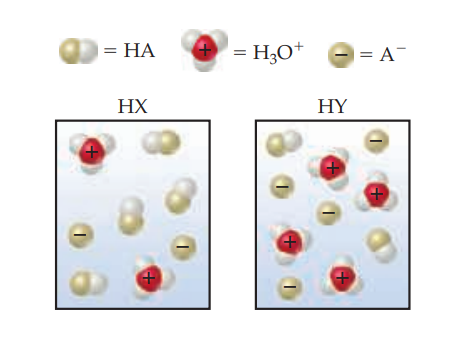×
Get Full Access to Chemistry: The Central Science - 14 Edition - Chapter 16 - Problem 16.2
Get Full Access to Chemistry: The Central Science - 14 Edition - Chapter 16 - Problem 16.2

×

# ?The following diagrams represent aqueous solutions of two monoprotic acids, HA (A = X or Y). The water molecules have been omitted for clarity.ISBN: 9780134414232 1274

## Solution for problem 16.2 Chapter 16

Chemistry: The Central Science | 14th Edition

• Textbook Solutions
• 2901 Step-by-step solutions solved by professors and subject experts
• Get 24/7 help from StudySoup virtual teaching assistantsChemistry: The Central Science | 14th Edition

4 5 1 271 Reviews
16
2
Problem 16.2

The following diagrams represent aqueous solutions of two monoprotic acids, HA (A = X or Y). The water molecules have been omitted for clarity.

(a) Which is the stronger acid, HX or HY?

(b) Which is the stronger base, $$\mathrm{X}^{-}$$ or $$\mathrm{Y}^{-}$$?

(c) If you mix equal concentrations of HX and NaY, will the equilibrium

$$\mathrm{HX}(a q)+\mathrm{Y}^{-}(a q) \rightleftharpoons \mathrm{HY}(a q)+\mathrm{X}^{-}(a q)$$

lie mostly to the right $$\left(K_{c}>1\right)$$ or to the left $$\left(K_{c}<1\right)$$? [Section 16.2]Text Transcription:

X-

Y-

HX(aq) + Y-(aq) rightleftharpoons HY(aq) + X-(aq)

(Kc > 1)

(Kc < 1)

Step-by-Step Solution:

Step 1 of 5) As an example, let’s consider a process involving the transfer of heat. When two objects at different temperatures are in contact, heat flows spontaneously from the hotter object to the colder one. Because it is impossible to make the heat flow in the opposite direction, from colder to hotter, this is an irreversible process. Given this fact, can we imagine any hypothetical conditions under which the heat transfer could be reversible The answer is yes, but only if we consider temperature changes that are infinitesimally small. Imagine the system and its surroundings at essentially the same temperature, with just an infinitesimal temperature difference, dT, between them (Figure 19.3). If the surroundings are at temperature T and the system is at an infinitesimally higher temperature T + dT, then an infinitesimal amount of heat flows from the system to surroundings. We can reverse the direction of heat flow by making an infinitesimal change of temperature in the opposite direction, lowering the system temperature to T - dT. Now the direction of heat flow is from surroundings to system. Thus, for the process to be reversible, the amounts of heat must be infinitesimally small and the transfer of heat must occur infinitely slowly.

Step 2 of 2

## Discover and learn what students are asking

Unlock Textbook Solution